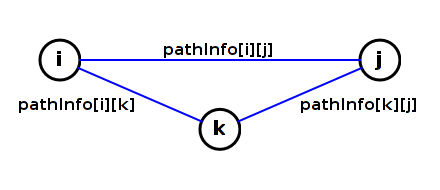#### The All-Pairs Shortest Paths Problem

The all-pairs shortest paths (APSP) problem is to find shortest paths between all pairs of vertices in a graph. There is a simple form for the solution that records a minimal amount of information needed to lookup distances along shortest paths as well as reconstruct the shortest paths.

There are some simple but inefficient algorithms to solve the APSP problem. The Floyd-Warshall agorithm gives a more efficient solution.

##### Assumption

For this presentation it is assumed that all edge weights are non-negative.

If the vertices in the graph are identified with integers then the solution to the APSP problem for the graph can be given in the form of a `pathInfo` matrix. Each entry `pathInfo[i][j]` is an object with two attributes:

• distance - the length of a shortest path from vertex i to vertex j
• neighbor - the neighbor of i on a shortest path from vertex i to vertex j

This matrix lets us quickly determine the shortest-path distance from a vertex i to a vertex j. To find the path itself, you first get `k1 = pathInfo[i][j].neighbor`. This gives you the first step on the path. The next step is to `k2 = pathInfo[k1][j].neighbor`. This is repeated until you reach `j`.

There are two inefficient ways of solving the APSP:

• Run a single-source shortest paths (SSSP) algorithm from each vertex. This has run time O(V*E*log(E)). If the graph has a lot of edges (that is E = Ω(V2)) then the run time is O(V3*log(V)).
• Use a variation of a matrix multiplication algorithm to compute the (V - 1)-st power of the adjacency matrix. This can be done with O(lg(V)) matrix "multiplications", each taking O(V3) time. Then the overall run time is again O(V3*log(V)).

The Floyd-Warshall algorithm

```      initialize pathInfo matrix using only paths with no intermediate vertices
for each vertex k
// Invariant:
// For every i and j, pathInfo[i][j] describes the shortest path from
// i to j among paths whose intermediate vertices are all less than k.
for each vertex i
for each vertex j
update pathInfo[i][j] to allow for using k as an intermediate vertex
end for
end for
end for
```

The following is the invariant for the outermost loop:

For every i and j, pathInfo[i][j] describes the shortest path from i to j among paths whose intermediate vertices are all less than k.

Initialization code should be designed to establish the invariant for k = 0. In other words, `pathInfo[i][j]` initially describes the shortest path from i to j that has no intermediate vertices. The paths we are considering have at most one edge.

If `i == j` (no edges) then

• `pathInfo[i][i].distance` should be set to `0` and
• `pathInfo[i][i].neighbor` should be set to `null`.

If there is an edge of weight `w` from vertex `i` to vertex `j` (one edge) then

• `pathInfo[i][j].distance` should be set to `w` and
• `pathInfo[i][j].neighbor` should be set to `j`.

Otherwise

• `pathInfo[i][j].distance` should be set to `Double.POSITIVE_INFINITY` and
• `pathInfo[i][j].neighbor` should be set to `null`.

#### Floyd-Warshall Update

The following is the invariant for the outermost loop:

For every i and j, pathInfo[i][j] describes the shortest path from i to j among paths whose intermediate vertices are all less than k.

Update code assumes the invariant is true for the current value of k. The code should be designed to establish the invariant for k + 1. This ensures that the invariant is true at the beginning of the next iteration of the outermost loop.

The update is suggested by the following diagram.Here, we are trying to update `pathInfo[i][j]`.

If `pathInfo[i][j].distance` < `pathInfo[i][k].distance` + `pathInfo[k][j].distance` then allowing `k` to be used as an intermediate vertex yields a shorter path. Then the update should change `pathInfo[i][j]`:

• `pathInfo[i][j].distance` is set to `pathInfo[i][k].distance + pathInfo[k][j].distance`
• `pathInfo[i][j].neighbor` is set to `pathInfo[i][k].neighbor`

Otherwise `pathInfo[i][j]` should not be changed.

Note that following these rules does not lead to an update of either `pathInfo[i][k]` or `pathInfo[k][j]`. The loop does not need special case code to deal with these cases.

#### Floyd-Warshall Run Time

The Floyd-Warshall run time is O(V3).